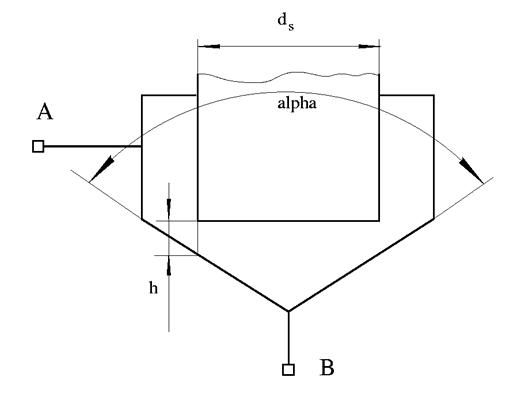# Poppet Valve

Hydraulic poppet valve

## Library

Flow Control Valves

•## Description

The Poppet Valve block models a variable orifice created by a cylindrical sharp-edged stem and a conical seat.The flow rate through the valve is proportional to the valve opening and to the pressure differential across the valve. The flow rate is determined according to the following equations:

`$q={C}_{D}\cdot A\left(h\right)\sqrt{\frac{2}{\rho }}\frac{\Delta p}{{\left(\Delta {p}^{2}+{p}_{\text{Cr}}^{2}\right)}^{1/4}},$`
`$\Delta p={p}_{\text{A}}-{p}_{\text{B}},$`
`$h={x}_{0}+x$`
`${h}_{\mathrm{max}}={d}_{s}\frac{\sqrt{1+\mathrm{cos}\frac{\alpha }{2}}-1}{\mathrm{sin}\alpha }$`

`${A}_{\mathrm{max}}=\frac{\pi {d}_{s}^{2}}{4}$`

where

 q Flow rate p Pressure differential pA, pB Gauge pressures at the block terminals CD Flow discharge coefficient A(h) Instantaneous orifice passage area x0 Initial opening x Stem displacement from initial position h Valve opening hmax Maximum valve opening. The passage area remains constant and equal to `Amax` after this. ds Stem diameter α Cone angle ρ Fluid density Aleak Closed valve leakage area Amax Maximum valve open area pcr Minimum pressure for turbulent flow

The minimum pressure for turbulent flow, pcr, is calculated according to the laminar transition specification method:

• By pressure ratio — The transition from laminar to turbulent regime is defined by the following equations:

pcr = (pavg + patm)(1 – Blam)

pavg = (pA + pB)/2

where

 pavg Average pressure between the block terminals patm Atmospheric pressure, 101325 Pa Blam Pressure ratio at the transition between laminar and turbulent regimes (Laminar flow pressure ratio parameter value)
• By Reynolds number — The transition from laminar to turbulent regime is defined by the following equations:

`${p}_{cr}=\frac{\rho }{2}{\left(\frac{{\mathrm{Re}}_{cr}\cdot \nu }{{C}_{D}\cdot {D}_{H}}\right)}^{2}$`
`${D}_{H}=\sqrt{\frac{4A}{\pi }}$`

where

 DH Valve instantaneous hydraulic diameter ν Fluid kinematic viscosity Recr Critical Reynolds number (Critical Reynolds number parameter value)

The block positive direction is from port A to port B. This means that the flow rate is positive if it flows from A to B and the pressure differential is determined as $\Delta p={p}_{\text{A}}-{p}_{\text{B}},$. Positive signal at the physical signal port `S` opens the valve.

## Basic Assumptions and Limitations

• Fluid inertia is not taken into account.

• The flow passage area is assumed to be equal to the frustum side surface area.

## Parameters

Valve stem diameter

The diameter of the valve stem. The default value is `0.01` m.

Seat cone angle

The angle of the valve conical seat. The parameter value must be in the range between 0 and 180 degrees. The default value is `120` degrees.

Initial opening

The initial opening of the valve. The parameter value must be nonnegative. The default value is `0`.

Flow discharge coefficient

Semi-empirical parameter for valve capacity characterization. Its value depends on the geometrical properties of the orifice, and usually is provided in textbooks or manufacturer data sheets. The default value is `0.65`.

Laminar transition specification

Select how the block transitions between the laminar and turbulent regimes:

• `Pressure ratio` — The transition from laminar to turbulent regime is smooth and depends on the value of the Laminar flow pressure ratio parameter. This method provides better simulation robustness.

• `Reynolds number` — The transition from laminar to turbulent regime is assumed to take place when the Reynolds number reaches the value specified by the Critical Reynolds number parameter.

Laminar flow pressure ratio

Pressure ratio at which the flow transitions between laminar and turbulent regimes. The default value is `0.999`. This parameter is visible only if the Laminar transition specification parameter is set to `Pressure ratio`.

Critical Reynolds number

The maximum Reynolds number for laminar flow. The value of the parameter depends on the orifice geometrical profile. You can find recommendations on the parameter value in hydraulics textbooks. The default value is `10`. This parameter is visible only if the Laminar transition specification parameter is set to `Reynolds number`.

Leakage area

The total area of possible leaks in the completely closed valve. The main purpose of the parameter is to maintain numerical integrity of the circuit by preventing a portion of the system from getting isolated after the valve is completely closed. The parameter value must be greater than 0. The default value is `1e-12` m^2.

## Global Parameters

Parameters determined by the type of working fluid:

• Fluid density

• Fluid kinematic viscosity

Use the Hydraulic Fluid block or the Custom Hydraulic Fluid block to specify the fluid properties.

## Ports

The block has the following ports:

`A`

Hydraulic conserving port associated with the valve inlet.

`B`

Hydraulic conserving port associated with the valve outlet.

`S`

Physical signal port to control spool displacement.

## Version History

Introduced in R2006a